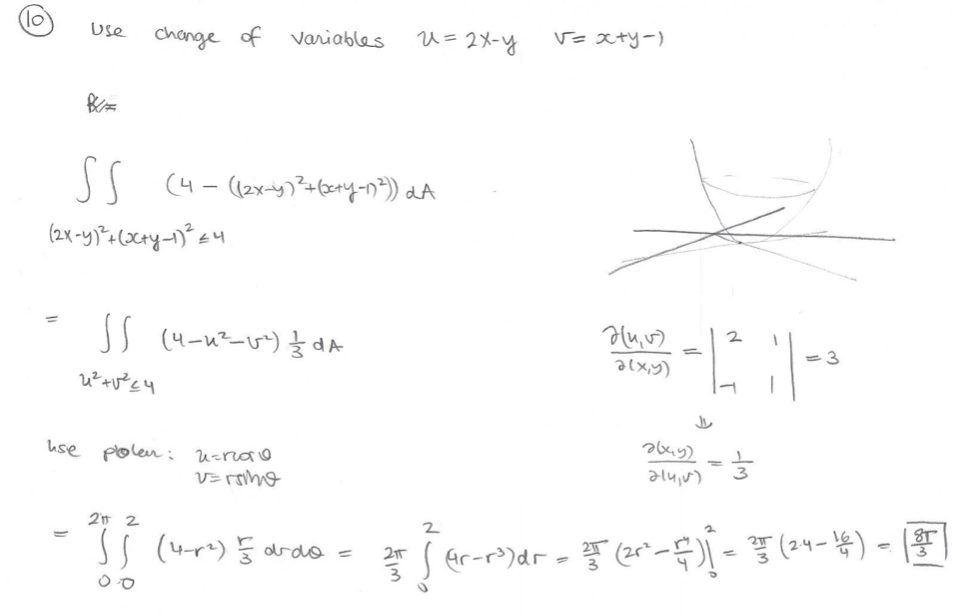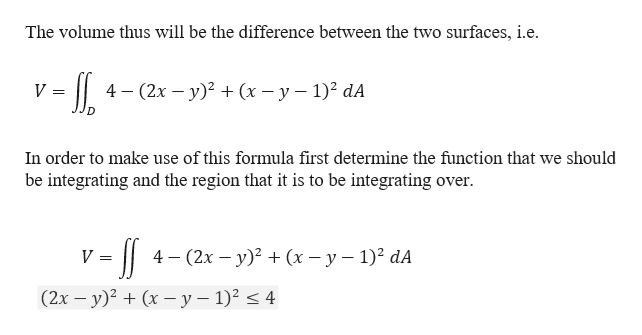# V= xty-)=2X-yUsechange of VariablesSS(2x-y (Xty-4-11S4-d3use polen; u-rnaoVrmhg2 2(4r rdeGr-r>)dr21T

Question
14 views

Find the volume of the solid bound by the surface z=(2x-y)^2+(x-y-1)^2 and the plane z=4.

Question: Why do we need to use the change of variables in this equation? How do we know when to use the change of variables?help_outlineImage TranscriptioncloseV= xty-) =2X-y Use change of Variables SS (2x-y (Xty-4 -11 S4- d 3 use polen; u-rnao Vrmhg 2 2 (4r rde Gr-r>)dr 21T fullscreen
check_circle

Step 1

Given that a solid is bounded by the surface and plane.

Step 2

To determine th...help_outlineImage TranscriptioncloseThe volume thus will be the difference between the two surfaces, i.e 4 - (2х — у)? + (х - у — 1)? dA V In order to make use of this formula first determine the function that we should be integrating and the region that it is to be integrating over 4 — (2х — у)? + (х — у — 1)? dA V = (2x - y)2 (x -y - 1) 4 fullscreen

### Want to see the full answer?

See Solution

#### Want to see this answer and more?

Solutions are written by subject experts who are available 24/7. Questions are typically answered within 1 hour.*

See Solution
*Response times may vary by subject and question.
Tagged in

### Calculus